Shape Names – Shape Vocabulary in English

Learn Shape Names in English – Shape Names – Shape Vocabulary in English. English shapes for kids;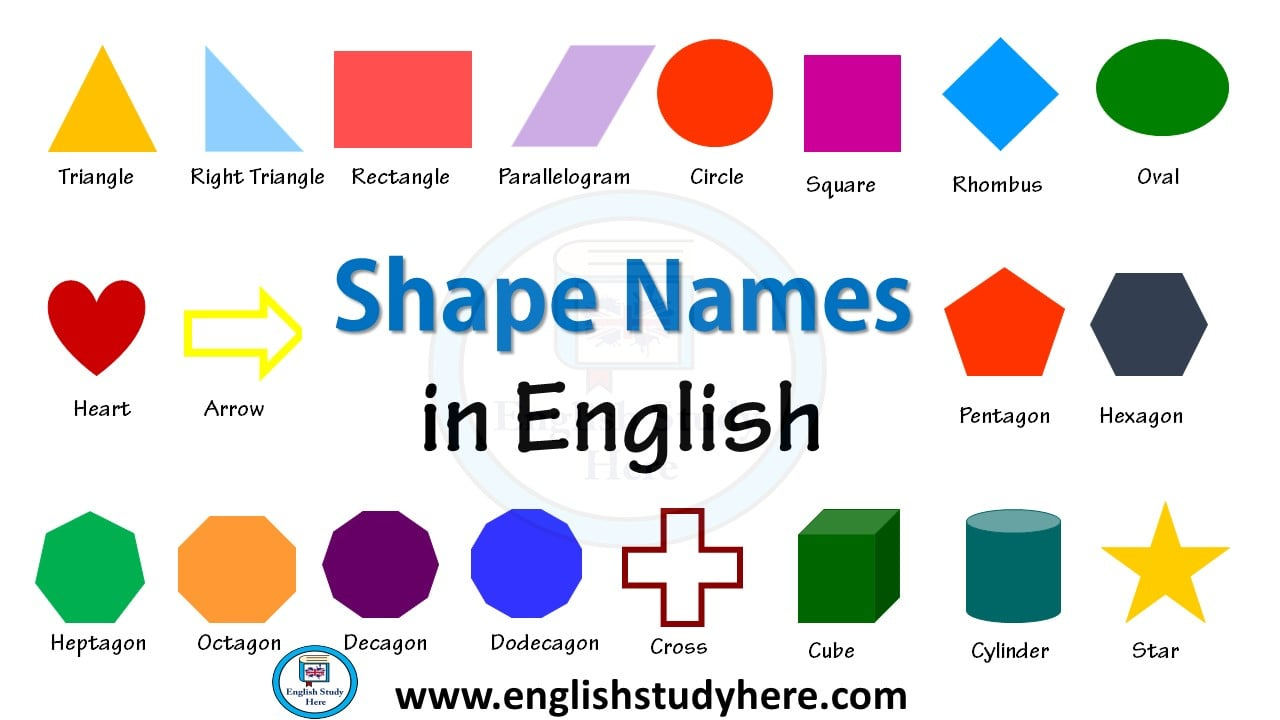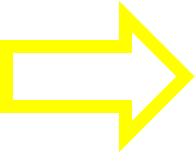Arrow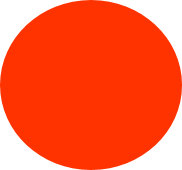Circle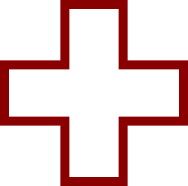Cross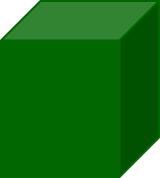Cube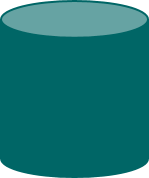CylinderDecagon

A polygon with nine sides and ten anglesDodecagon

A polygon with nine sides and twelve anglesHeart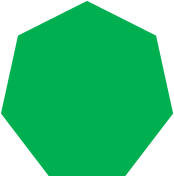Heptagon

A polygon with nine sides and seven anglesHexagon

A polygon with nine sides and six angles.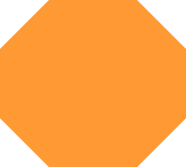Octagon

A polygon with nine sides and eight angles.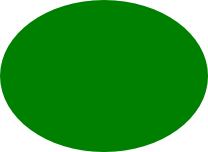Oval

A shape rather like an egg or an ellipse.Parallelogram

A convex quadrilateral in which each pair of opposite edges are parallel and of equal length.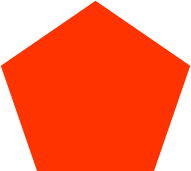Pentagon

A polygon with nine sides and five angles.Rectangle

It has four right angles.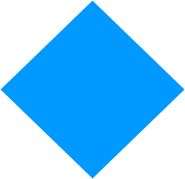Rhombus

Having all sides of equal length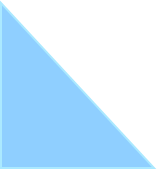Right TriangleSquare

The interior angles of a square are right angles or angles of 90 degrees.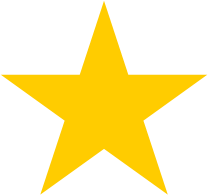StarTriangle

A polygon with nine sides and three angles.